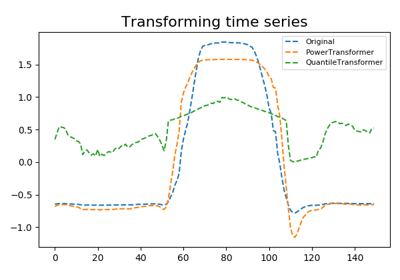# `pyts.preprocessing`.QuantileTransformer¶

class `pyts.preprocessing.``QuantileTransformer`(n_quantiles=1000, output_distribution='uniform', subsample=100000, random_state=None)[source]

Transform samples using quantiles information.

This method transforms the samples to follow a uniform or a normal distribution. Therefore, for a given sample, this transformation tends to spread out the most frequent values. It also reduces the impact of (marginal) outliers: this is therefore a robust preprocessing scheme. The transformation is applied on each sample independently.

The cumulative distribution function of a feature is used to project the original values. Note that this transform is non-linear.

Parameters: n_quantiles : int, optional (default = 1000) Number of quantiles to be computed. It corresponds to the number of landmarks used to discretize the cumulative distribution function. output_distribution : ‘uniform’ or ‘normal’ (default = ‘uniform’) Marginal distribution for the transformed data. The choices are ‘uniform’ (default) or ‘normal’. subsample : int, optional (default = 1e5) Maximum number of timestamps used to estimate the quantiles for computational efficiency. random_state : int, RandomState instance or None, optional (default=None) If int, random_state is the seed used by the random number generator; If RandomState instance, random_state is the random number generator; If None, the random number generator is the RandomState instance used by np.random. Note that this is used by subsampling and smoothing noise.

Examples

```>>> from pyts.datasets import load_gunpoint
>>> from pyts.preprocessing import QuantileTransformer
>>> X, _, _, _  = load_gunpoint(return_X_y=True)
>>> qt = QuantileTransformer(n_quantiles=10)
>>> qt.transform(X) # doctest: +ELLIPSIS
array([...])
```

Methods

 `__init__`(self[, n_quantiles, …]) Initialize self. `fit`(self[, X, y]) Pass. `fit_transform`(self, X[, y]) Fit to data, then transform it. `get_params`(self[, deep]) Get parameters for this estimator. `set_params`(self, \*\*params) Set the parameters of this estimator. `transform`(self, X) Transform the data.
`__init__`(self, n_quantiles=1000, output_distribution='uniform', subsample=100000, random_state=None)[source]

Initialize self. See help(type(self)) for accurate signature.

`fit`(self, X=None, y=None)[source]

Pass.

Parameters: X Ignored y Ignored self : object
`fit_transform`(self, X, y=None, **fit_params)

Fit to data, then transform it.

Fits transformer to X and y with optional parameters fit_params and returns a transformed version of X.

Parameters: X : numpy array of shape [n_samples, n_features] Training set. y : numpy array of shape [n_samples] Target values. **fit_params : dict Additional fit parameters. X_new : numpy array of shape [n_samples, n_features_new] Transformed array.
`get_params`(self, deep=True)

Get parameters for this estimator.

Parameters: deep : bool, default=True If True, will return the parameters for this estimator and contained subobjects that are estimators. params : mapping of string to any Parameter names mapped to their values.
`set_params`(self, **params)

Set the parameters of this estimator.

The method works on simple estimators as well as on nested objects (such as pipelines). The latter have parameters of the form `<component>__<parameter>` so that it’s possible to update each component of a nested object.

Parameters: **params : dict Estimator parameters. self : object Estimator instance.
`transform`(self, X)[source]

Transform the data.

Parameters: X : array-like, shape = (n_samples, n_timestamps) Data to transform. X_new : array-like, shape = (n_samples, n_timestamps) Transformed data.

## Examples using `pyts.preprocessing.QuantileTransformer`¶Transformers